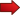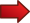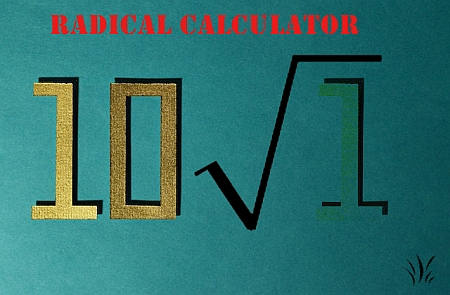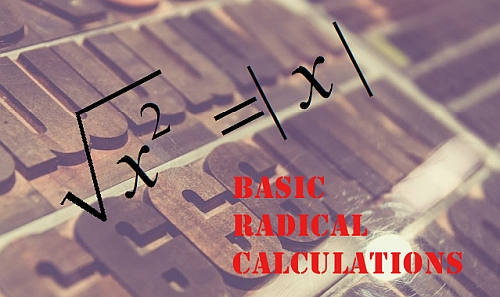Algebraic expressions containing radicals are very common, and it is important to know how to correctly handle them. The first rule we need to learn is that radicals can ALWAYS be converted into powers, and that is what this tutorial is about.In this tutorial we are going to learn how to simplify radicals.

Indeed, we deal with radicals all the time, especially with $$\sqrt x$$. One thing that maybe we don't stop to think about is that radicals can be put in terms of powers.

How do I do so? Check it out. Let us start with $$\sqrt x$$ first:

$\large \boxed{\sqrt x = x^{1/2}}$So why we should be excited about the fact that radicals can be put in terms of powers??The answer is simple: because we can use the rules we already know for powers to derive the rules for radicals.

For example, let $$x, y\ge 0$$ be two non-negative numbers. One rule that applies to radicals is

$\large \sqrt{x \cdot y} = \sqrt{x} \cdot \sqrt{y}$

How do we know? Well, simply by using rule 6 of exponents and the definition of radical as a power. Check it out:

$\large \sqrt{x\cdot y} = (x \cdot y)^{1/2} = x^{1/2} \cdot y^{1/2} = \sqrt{x} \cdot \sqrt{y}$

### EXAMPLE 1: Simplify the following radical expression:

$\large \displaystyle \sqrt{27x^5 y^7}$

Based on the given expression given, we can rewrite the elements inside of the radical to get

$\large \displaystyle \sqrt{27x^5 y^7} = \sqrt{3^3 x^4 \cdot x y^6 \cdot y}$ $\large \displaystyle = \sqrt{3^2 \cdot 3 x^4 \cdot x y^6 \cdot y}$ $\large \displaystyle = \sqrt{(3^2 x^4 y^6) \cdot (3x y)}$ $\large \displaystyle = \sqrt{3^2 x^4 y^6} \sqrt{3xy}$ $\large \displaystyle = \sqrt{3^2}\sqrt{x^4}\sqrt{ y^6} \sqrt{3xy}$ $\large \displaystyle = 3x^2y^3 \sqrt{3xy}$There are rules for operating radicals that have a lot to do with the exponential rules (naturally, because we just saw that radicals can be expressed as powers, so then it is expected that similar rules will apply).

Rule 1: $$\large \displaystyle \sqrt{x^2} = |x|$$

Rule 2: $$\large\displaystyle \sqrt{xy} = \sqrt{x} \sqrt{y}$$

Rule 3: $$\large\displaystyle \sqrt{\frac{x}{y}} = \frac{\sqrt x}{\sqrt y}$$

Most likely you have, one way or the other worked with these rules, sometimes even not knowing you were using them.

One specific mention is due to the first rule. Often times, you will see (or even your instructor will tell you) that $$\sqrt{x^2} = x$$, with the argument that the "root annihilates the square". To a degree, that statement is correct, but it is not true that $$\sqrt{x^2} = x$$. Indeed, we can give a counter example: $$\sqrt{(-3)^2} = \sqrt(9) = 3$$. So in this case, $$\sqrt{x^2} = -x$$.

In reality, what happens is that $$\sqrt{x^2} = |x|$$. This is the case when we get $$\sqrt{(-3)^2} = 3$$, because $$|-3| = 3$$.

### EXAMPLE 2

$\large \displaystyle \sqrt{\frac{8 x^5 y^6}{5 x^8 y^{-2}}}$

There are several things that need to be done here. First, we see that this is the square root of a fraction, so we can use Rule 3. Then, there are negative powers than can be transformed.

Concretely, we can take the $$y^{-2}$$ in the denominator to the numerator as $$y^2$$. Then, we can simplify some powers So we get:

$\large \displaystyle \sqrt{\frac{8 x^5 y^6}{5 x^2 y^{-2}}} = \sqrt{\frac{8 x^5 y^6 y^{2}}{5 x^8 }}$ $\large \displaystyle = \sqrt{\frac{2^3 y^{6+2}}{5 x^{8-5} }} = \sqrt{\frac{2^3 y^8}{5 x^3 }}$ $\large \displaystyle = \frac{\sqrt{2^3 y^8}}{\sqrt{5 x^3 }} = \frac{2y^4 \sqrt{2}}{x\sqrt{5 x }}$Observe that we analyzed and talked about rules for radicals, but we only consider the square root $$\sqrt x$$. Question is, do the same rules apply to other radicals (that are not the square root)? Short answer: Yes

Just to have a complete discussion about radicals, we need to define radicals in general, using the following definition:

$\large \boxed{\sqrt[n] x = x^{1/n}}$

With this definition, we have the following rules:

Rule 1.1: $$\large \displaystyle \sqrt[n]{x^n} = x$$, when $$n$$ is odd.

Rule 1.2: $$\large \displaystyle \sqrt[n]{x^n} = |x|$$, when $$n$$ is even.

Rule 2: $$\large\displaystyle \sqrt[n]{xy} = \sqrt[n]{x} \sqrt[n]{y}$$

Rule 3: $$\large\displaystyle \sqrt[n]{\frac{x}{y}} = \frac{\sqrt[n]{x}}{\sqrt[n]{y}}$$